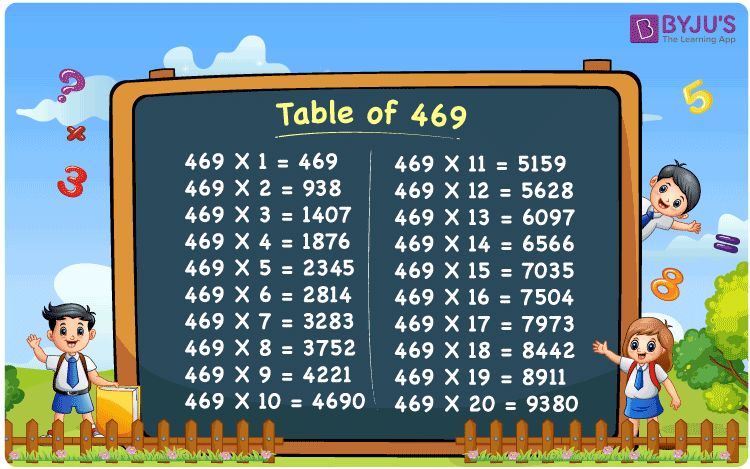Checkout JEE MAINS 2022 Question Paper Analysis : Checkout JEE MAINS 2022 Question Paper Analysis :

# Table of 469

The Table of 469 is given here to help students learn the quick calculation of problems. 469 times table produces the multiples of 469. The table of 469 can be formulated by multiplying 469 with natural numbers and by the constant addition of 469 to which it is multiplied. For example, 469 x 4 = 469 + 469 + 469 + 469 = 1876. Similarly, we can write the multiples of 469 for 20 times. Learn more multiplication tables only at BYJU’S. Also, access the table of 469 in PDF format, for free.## What is the 469 Times Table?

Learn how to write the multiplication table of 469 using multiplication and addition form from the chart given below.

 469×1 = 469 469 469×2 = 938 469 + 469 = 938 469×3 = 1407 469 + 469 + 469 = 1407 469×4 = 1876 469 + 469 + 469 + 469 = 1876 469×5 = 2345 469 + 469 + 469 + 469 + 469 = 2345 469×6 = 2814 469 + 469 + 469 + 469 + 469 + 469 = 2814 469×7 = 3283 469 + 469 + 469 + 469 + 469 + 469 + 469 = 3283 469×8 = 3752 469 + 469 + 469 + 469 + 469 + 469 + 469 + 469 = 3752 469×9 = 4221 469 + 469 + 469 + 469 + 469 + 469 + 469 + 469 + 469 = 4221 469×10 = 4690 469 + 469 + 469 + 469 + 469 + 469 + 469 + 469 + 469 + 469 = 4690

## Multiplication Table of 469

Learn the table of 469 for 20 results from the chart mentioned below.

 469 × 1 = 469 469 × 2 = 938 469 × 3 = 1407 469 × 4 = 1876 469 × 5 = 2345 469 × 6 = 2814 469 × 7 = 3283 469 × 8 = 3752 469 × 9 = 4221 469 × 10 = 4690 469 × 11 = 5159 469 × 12 = 5628 469 × 13 = 6097 469 × 14 = 6566 469 × 15 = 7035 469 × 16 = 7504 469 × 17 = 7973 469 × 18 = 8442 469 × 19 = 8911 469 × 20 = 9380

## Solved Example on the Table of 469

Example 1:

Evaluate 469 x 16 – 6000.

Solution:

From the table of 469,

469 x 16 = 7504.

7504 – 6000 = 1504.

## Frequently Asked Questions on Table of 469

### What is table of 469?

Table of 469 is the multiplication table that shows the product of the original number with natural numbers.

### Find 469 times 14 minus 50 plus 90.

469 times 14 = 469 x 14 = 6566. 469 times 14 minus 50 plus 90 = 6566 – 50 + 90 = 6606.

### Is 469 a composite number?

Yes, 469 is a composite number because it has more than two factors.